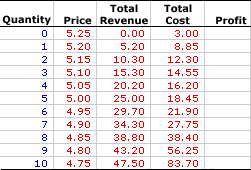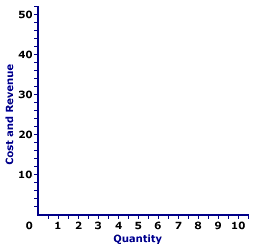Sunday  September 27, 2020
 AmosWEB means Economics with a Touch of Whimsy!DEMAND DETERMINANTS: Five basic ceteris paribus factors that affect demand, but which are assumed constant when a demand curve is constructed. Changes in any one causes a shift of the demand curve. The five demand determinants are: income, preferences, other prices, buyers' expectations, and number of buyers.MONOPOLISTIC COMPETITION, TOTAL ANALYSIS:

A monopolistically competitive firm produces the profit-maximizing quantity of output that generates the greatest difference between total revenue and total cost. This total approach is one of three methods that used to determine the profit-maximizing quantity of output. The other two methods involve the direct analysis of economic profit and a comparison of marginal revenue and marginal cost.
Monopolistic competition is a market structure with a large number of relatively small firms that sell similar but not identical products. Each firm is small relative to the overall size of the market such that it has some market control, but not much. In other words, it can sell a wide range of output at a narrow range of prices. This translates into a relatively elastic demand curve. If a monopolistically competitive firm wants to sell a larger quantity, then it must lower the price.

Comparable to any profit-maximizing firm, a monopolistically competitive firm produces the quantity of output in the short run that maximizes the difference between total revenue with total cost, which is economic profit. At this production level, the firm cannot increase profit by changing the level of production. The analysis of total revenue and total cost can be achieved through a table of numbers or with total revenue and total cost curves.

### Working the Numbers

A monopolistically competitive firm is presumed to produce the quantity of output that maximizes economic profit--the difference between total revenue and total cost. This decision can be analyzed using this table presented below. This table presents revenue and cost information for Manny Mustard's House of Sandwich, a hypothetical example of a monopolistically competitive firm, that results from the production and sale of Deluxe Club Sandwiches, a tasty luncheon consumed by residents of Shady Valley.

Because Manny produces a product with a large number of close but slightly different substitutes, he has some market control but not much. As such, he faces a negatively-sloped demand curve, but one that is relatively elastic. To sell a larger quantity, he must lower the price. Manny Mustard's status as a monopolistically competitive firm is reflected in this table.

The Sandwich Numbers• Quantity: The quantity of output produced by Manny Mustard, presented in the first column, ranges from 0 to 10 sandwiches. While, Manny Mustard could produce more than 10 sandwiches, this range is sufficient for the present analysis.

• Price: The second column presents the price received by Manny Mustard for selling sandwiches. As a price maker, the first and second columns represent the demand facing Manny for his sandwiches. The price Manny faces ranges from a high of \$5.25 per sandwich for a zero quantity to a low of \$4.75 per sandwich for 10 sandwiches. Manny Mustard can sell a larger quantity of sandwiches, but only by reducing the price. Manny Mustard is a price maker.

• Total Revenue: Total revenue is presented in the third column. This indicates the revenue Manny Mustard receives at each level of sandwich production. It is derived as the quantity in the first column multiplied by the price in the second column. Total revenue ranges from \$0 if no output is sold to a high of \$47.50 for selling 10 sandwiches. For example, selling 5 sandwiches generates \$25 of revenue and selling 8 sandwiches leads to \$38.80 of revenue.

• Total Cost: The fourth column presents the total cost incurred by Manny in the production of his sandwiches, ranging from a low of \$3 for zero output (which is fixed cost) to a high of \$83.70 for 10 sandwiches. Total cost continues to rise beyond 10 sandwiches, but this information is not needed for the present analysis. Producing 1 sandwich incurs a total cost of \$8.85. Producing 2 sandwiches incurs a total cost of \$12.30. Total cost rises as Manny produces more.

• Profit: The fifth column at the far right of the table is available to display economic profit, the difference between total revenue in the third column and total cost in the fourth column. A click of the [Profit] button displays Manny's economic profit in the fifth column.
The task is to identify the quantity of sandwich production that provides the maximum profit. A consideration of the options is in order.

A click of the [Incurring Losses] button indicates that producing and selling 1 sandwich generates an economic loss of \$3.65. Total revenue is \$5.20 and total cost is \$8.85. A \$2 loss results from 2 sandwiches. Manny incurs an economic loss for the first 2 sandwiches. Manny also incurs an economic loss if production is 9 sandwiches or more.

But loss is not what Manny Mustard seeks. Click the [Earning Profits] button to highlight the range of production levels that generate positive economic profit. Manny initially turns his profit picture around with 3 sandwiches. At 3 sandwiches Manny's total revenue is greater than his total cost by \$0.75. Profit remains positive through the production of 8 sandwiches as Manny's total revenue exceeds his total cost. For 5 sandwiches, this profit is \$6.55, for 6 profit is \$7.80, and for 7 profit drops back to \$6.55 again.

So what is the profit-maximizing level of sandwich production Manny should undertake? The desired production level is clearly not 2 sandwiches or less, nor is it 9 sandwiches or more, all of which lead to economic loss. It must be within the highlighted range from 3 to 8 sandwiches.

The quantity that generates the greatest level of economic profit is 6 sandwiches. This alternative can be highlighted by clicking the [Profit Max] button. The production of 6 sandwiches results in \$29.70 of total revenue and \$21.90 of total cost, a difference of \$7.80. No other production level generates a greater economic profit. Producing 1 more sandwich or 1 less sandwich reduces profit to \$6.55.

### Working the Curves

The Sandwich CurvesThe short-run production decision for a monopolistically competitive firm can be graphically illustrated using total revenue and total cost curves. The exhibit to the right is standing poised to display these curves.
• Total Revenue: A click of the [Total Revenue] button reveals a slightly curved green line labeled TR that depicts the total revenue that Manny Mustard receives from sandwich production and sales. The line is curved because Manny Mustard faces a relatively elastic, negatively-sloped demand curve.

• Total Cost: A click of the [Total Cost] button reveals a more curvy red line labeled TC represents the total cost Manny Mustard incurs in the production of sandwiches. The shape is based on increasing, then decreasing marginal returns.

• Profit: The vertical difference between these two lines is economic profit. Where the total revenue line lies above the total cost line in the middle of the diagram, economic profit is positive. Where the total revenue line lies below the total cost line at the far right and far left, economic profit is negative.
The key for Manny Mustard is to identify the production level that gives the greatest vertical distance between the total revenue and total cost curves in the middle of the diagram. This might not be evident by just looking at the exhibit, but it can be illustrated by clicking the [Profit Max] button. The output quantity identified is, once again, 6 sandwiches.

Before leaving this graph, two other quantities can be highlighted. The total revenue and total cost curves intersect at two quantities--at just under 3 sandwiches and just over 8 sandwiches. Click the [Breakeven] button to highlight these two output levels.

Both quantities are termed breakeven output. Breakeven output is a quantity of output in which the total revenue is equal to total cost such that the firm earns exactly a normal profit, and thus receives no economic profit nor incurs an economic loss. The reason for the term "breakeven" output is that the firm is just "breaking even." It is neither making a profit nor incurring a loss. Economic profit is zero. Breakeven output is usually most noteworthy as a reference point. The profit-maximizing production level invariably occurs between the two breakeven output levels.

 <= MONOPOLISTIC COMPETITION, SHUTDOWN MONOPOLY =>Recommended Citation:

MONOPOLISTIC COMPETITION, TOTAL ANALYSIS, AmosWEB Encyclonomic WEB*pedia, http://www.AmosWEB.com, AmosWEB LLC, 2000-2020. [Accessed: September 27, 2020].

Check Out These Related Terms...

Or For A Little Background...

And For Further Study...
Search Again?BLACK DISMALAPOD[What's This?] Today, you are likely to spend a great deal of time waiting for visits from door-to-door solicitors trying to buy either a birthday greeting card for your grandfather or a weathervane with a cow on top. Be on the lookout for the happiest person in the room.Your Complete ScopeIt's estimated that the U.S. economy has about \$20 million of counterfeit currency in circulation, less than 0.001 perecent of the total legal currency."The way employees treat customers reflects the manner in which they're treated by management. "-- James Perkins, president, Cornell UniversityACRSAccelerated Cost Recovery SystemA PEDestrian's Guide Xtra CreditTell us what you think about AmosWEB. Like what you see? Have suggestions for improvements? Let us know. Click the User Feedback link.| | | | | | | | | | |
| | | |

Thanks for visiting AmosWEB